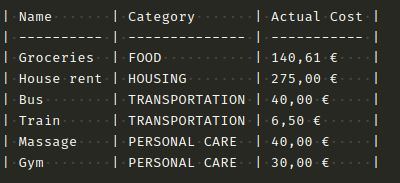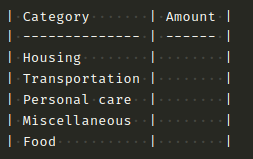# How can i get the sum of values for each category?

I have this table to register my expenses:I want other table where i can have the total amount for each category:What is the formula to get the total amount/cost for each category?

Example: `SUM("Actual Cost") where Category="TRANSPORTATION"`

Hi, Try using SUMIF, see attached file.LOQ20200607.ods

Also, this is a generic enough request that you can search for this solution for Excel that would work just as well in LibreOffice.

The easy way it’s with a pivot table.

Creating Pivot Tables